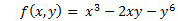# SQL Server HESSIAN function

HESSIAN
Updated: 15 April 2018

Use the scalar function HESSIAN to numerically compute the Hessian matrix. HESSIAN assumes that the function has continuous partial derivatives. HESSIAN produces a square matrix of second order partial derivatives of a scalar function.Syntax
SELECT [wct].[HESSIAN] (
<@Func, nvarchar(max),>
,<@VarNames, nvarchar(4000),>
,<@X, nvarchar(4000),>
,<@H, float,>)
Arguments
 Input Name Description @Func The function to be evaluated, as a string. The function must be in the form of a SELECT statement. @VarName The name of the variable @X The point where the Hessian is calculated @H Step size
Return Type
[nvarchar](max)
Remarks
• If @Func returns a NULL then NULL Is returned.
• If @Func is not a valid SELECT statement then NULL is returned.
• If no solution is found then NULL is returned.
• If @X is NULL then @X = ''.
• If @H is NULL then @H = 0.
• If @H <= 0 then @H = 2^(-13)
• Available in XLeratorDB / math 2008 only
Examples
Example #1

Calculate the Hessian for the functionAt the point (1,2)

Since the result is returned as a string, we will use the MATRIX function to unpack the results into a matrix format.

SELECT
*
FROM wctMath.wct.MATRIX(
wct.HESSIAN(
'SELECT POWER(@x,3) - 2*@x*@y - POWER(@y,6)'
,'@x,@y'
,'1,2'
,NULL
)
)

This produces the following result.Copyright 2008-2021 Westclintech LLC         Privacy Policy        Terms of Service